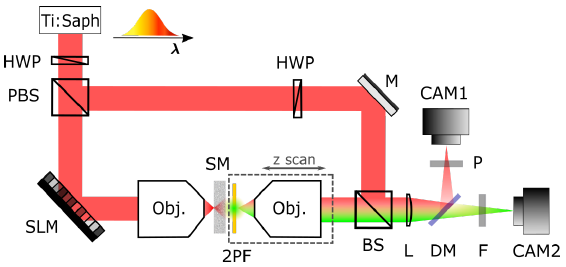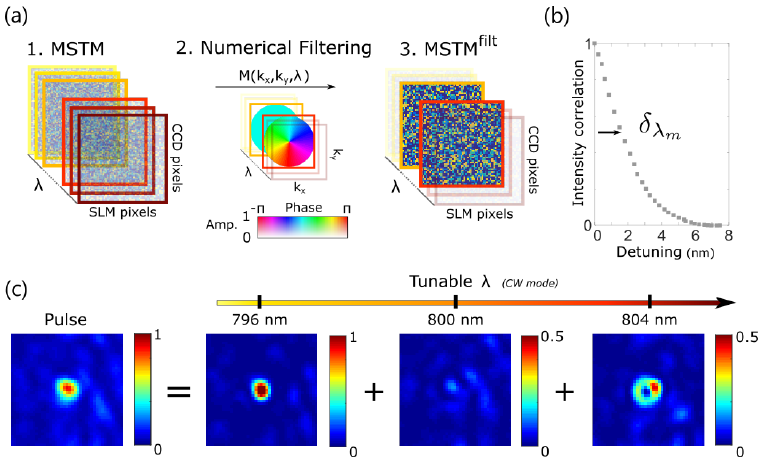## Spatio-temporal shaping of light through a scattering medium[A. Boniface et al., arxiv, 2007.09050 (2020)]

Controlling the spatial and temporal properties of a short pulse is already difficult in free space, and require a well-calibrated and specific setup. When the pulse propagates through a scattering medium, its temporal and spatial properties are randomized, leading to a spatio-temporal speckle. However, the effect of the medium can be described by means of the multi-spectral transmission matrix. In this paper, A. Boniface and his colleagues show that by measuring previously this matrix, and by only controlling the spatial input field using a spatial light modulator, they can modulate at will the spatio-temporal properties of the point spread function of a pulse traveling through a complex medium.

At a given frequency, light propagation between two planes through any linear system can be described by a matrix, the so-called transmission matrix. For disordered systems, like random scattering media, this transmission matrix is seemingly random, traducing the fact that an input coherent illumination produces at the output a speckle pattern resulting from the interference of many random paths. We can see this feature as a mixing of the spatial degrees of freedom. For such media, we typically have a short spectral correlation width $$\delta \lambda$$, meaning that two input frequencies separated by more than $$\delta \lambda$$ gives rise to two uncorrelated output speckle patterns. As a result, il we illuminate the medium with a short pulse and measure the intensity at any point at the output, the temporal signal is broadened and also looks like a random speckle. This is due to the fact that the spectral components of the field are not anymore in phase, but have acquired random phases, just like the spatial components.

For characterizing the propagation of a short pulse of bandwidth $$\Delta\lambda$$, it is then necessary to estimate the multi-spectral transmission matrix of the propagating medium, which is defined as a 3-dimension tensor :

$$H(\lambda,\mathbf{r}_{out},\mathbf{r}_{in})\tag{1}$$

It consists of the stack of transmission matrices for a set of uncorrelated input wavelengths inside the bandwidth of the pulse. This operator characterizes the mixing of the spatio-temporal degrees of freedom. In the paper, the authors use the setup presented in Figure 1. to measure the transmission matrix at different frequencies by sending a set of illumination patterns and measuring the corresponding complex output fields using a reference arm.Figure 1. Experimental setup. Image from [A. Boniface et al., arxiv, 2007.09050 (2020)]

Theoretically, knowing this multi-spectral transmission matrix, one can shape at will the output field in space and time (or equivalently spectrally). For instance, by putting all the spectral components in phase in one output point, one can obtain a sharp focus of light despite scattering, or focus different spectral components at different positions. However, taking full advantage of the multi-spectral transmission matrix requires to be able to control independently the spatial and temporal degrees of freedom, in other words, to be able to control the amplitude and the phase of every spectral component on each pixel, which is not realistic when controlling a high number of pixels. In the paper, a mask is displayed on a spatial light modulator which is illuminated by a short pulse, which means that each spectral (or temporal) component of the input field sees the same amplitude and phase mask.

In the general case, if one wants a given spatio-temporal field on the output, the optimal solution requires to control the spectral component of the fields on each pixel, which is out of reach here. However, despite this restriction, there is still a way to focus light in space and in time at a given output point $$\mathbf{r}_{target}$$. In such a case, we only need to control of the field at the target location, by putting all the spectral contributions phase. Because we restrict our constraints on the output field to only one location we can now use a phase-conjugation approach.

Since we fix the target location $$\mathbf{r}_{target}$$, $$H(\lambda,\mathbf{r}_{target},\mathbf{r}_{in})$$ is represented by a 2D matrix $$\mathbf{H}_{target}$$. Its components  $$H_{ij}$$ links the complex modulation on the input pixel $$j$$ to the $$i^{th}$$ spectral components of the field at the target point $$\mathbf{r}_{target}$$. Phase conjugation consists in sending the mask that compensates for the phase introduced by the propagation. To focus in time, we need to put the spectral components in phase, so our target output spectral field (at the target location) is represented by a constant value vector $$[1,1,\ldots,1]$$. The input mask to send is then:

\begin{equation}
E^{in} = \mathbf{H}_{target}^\dagger [1,1,\ldots,1]^t \label{eq:1}\tag{2}
\end{equation}

where $$^\dagger$$ represents the transpose conjugate.

Now, what if we want another point-spread function (PSF)? Again, we only control the spatial components of the input field, so we want to restrict the constraints on one output position, as before, to find a direct solution to the problem. The elegant solution proposed by the author (first introduced by the same group for purely spatial shaping in [A. Boniface, Optica, 4 (2017)]) consists, instead of changing the target, in modifying the multi-spectral transmission matrix so that it contains the properties of the PSF we want. If $$M(\mathbf{k}_x,\mathbf{k}_y,\lambda_i)$$ is the spatial Fourier transform of the wanted PSFs for each wavelength $$\lambda_i$$, we expressed our new filtered multi-spectral transmission matrix as:

$$H^{filt} = F^{-1} \times M \times F \times H$$

where $$F$$ represents the Fourier transform operator.

We can now use the same operation as in equation $$\ref{eq:1}$$ to find the input mask and obtain the desired PSF, but using $$H^{filt}$$ instead of $$H$$. The principle of the technique is summarized in Figure 2.a.Figure 2. Experimental results. (a) Principle of the experiment. (b) Spectral correlation. (c) Engineered multi-spectral PSF.

To demonstrate the principle, the authors show in Figure 2.c. a multi-spectral PSF that corresponds to two different PSFs at two different frequencies.

In the rest of the paper, the authors show two more examples of the usage of the proposed technique. The basic idea is that the degrees of freedom offered by wavefront shaping and the multi-spectral transmission matrix can be used to improve the spatial or spectral/temporal properties of the PSF compared to standard approaches.

Created by sebastien.popoff on 01/09/2020